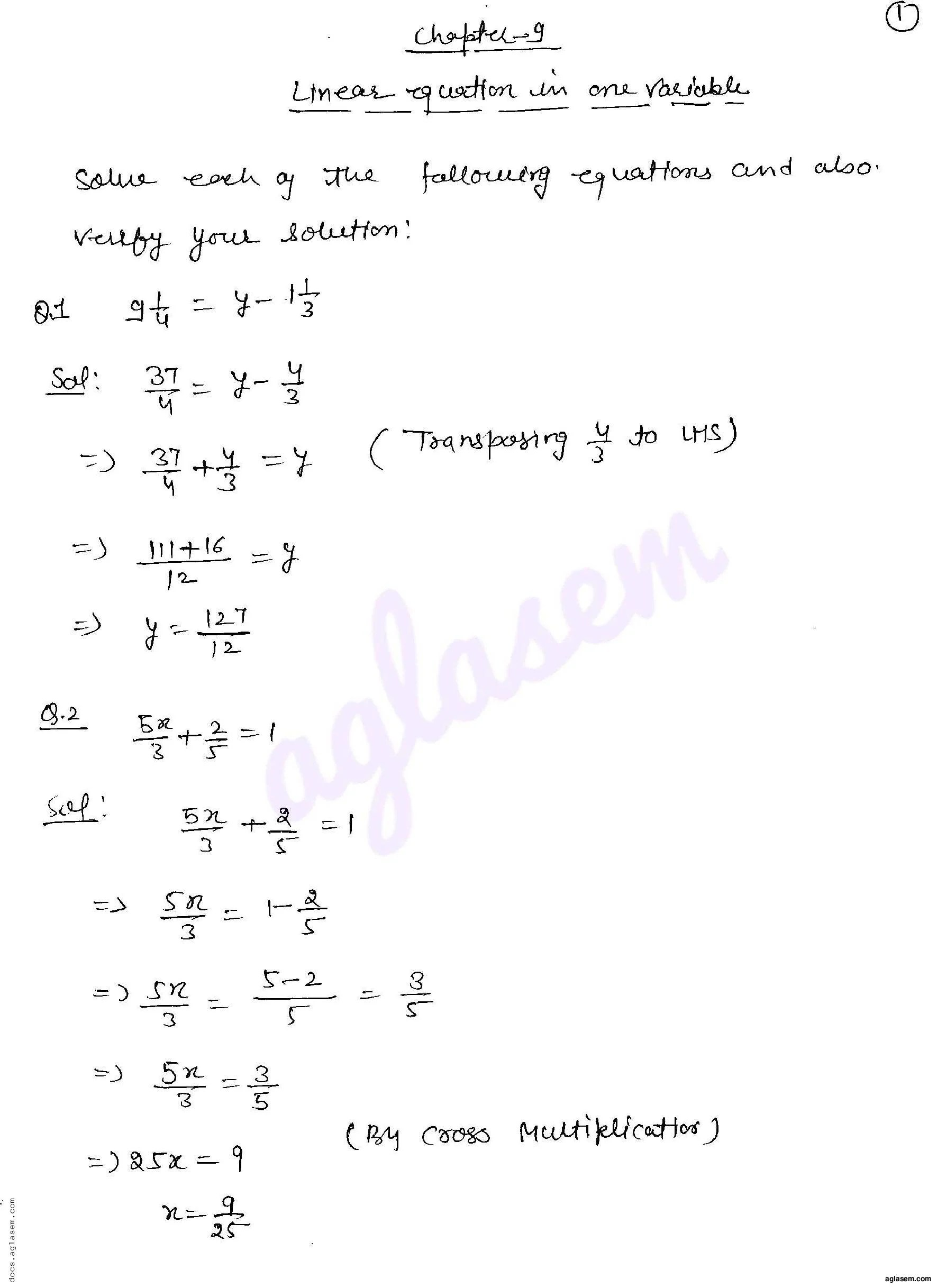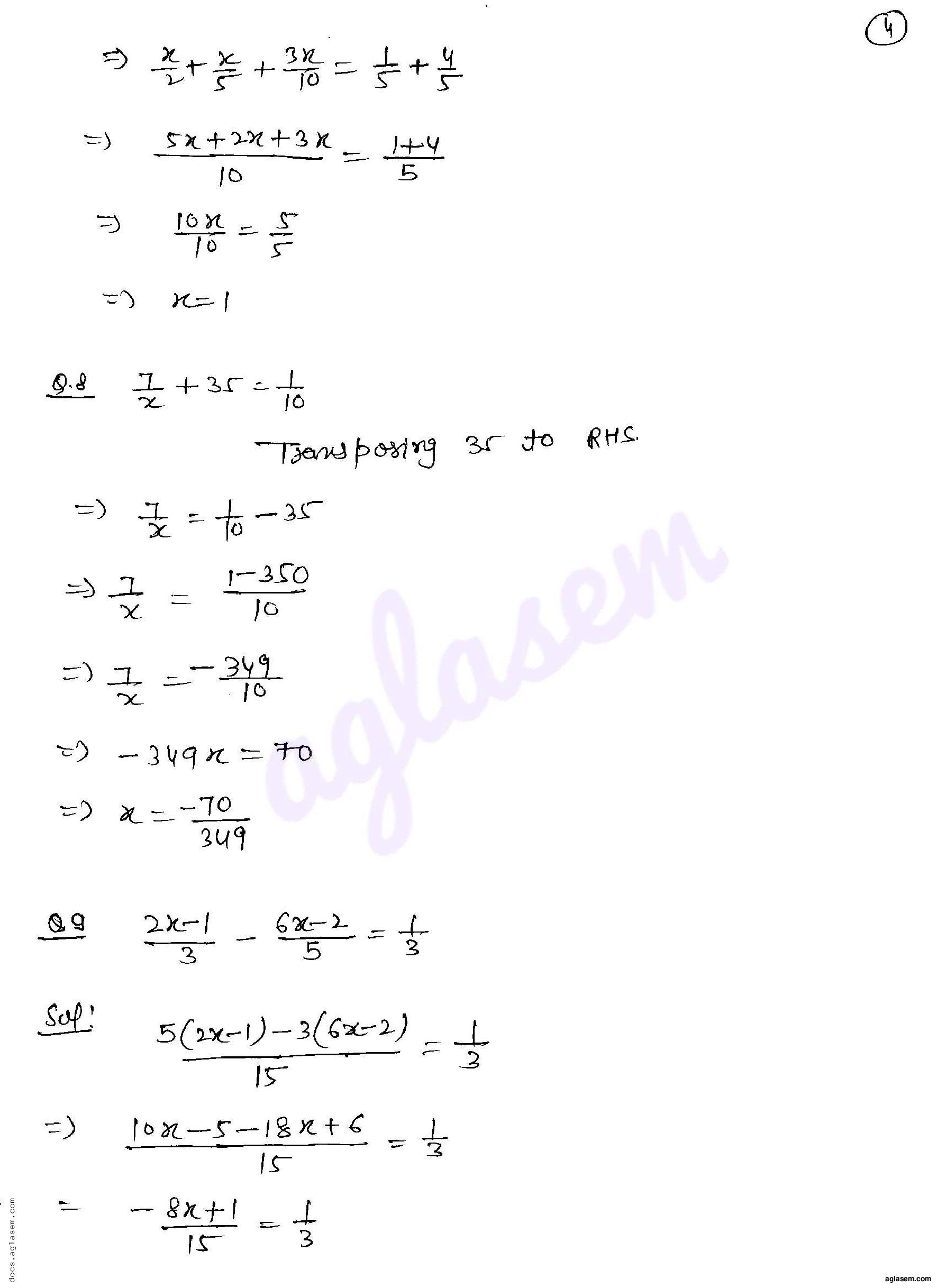# RD Sharma Solutions Class 8 Chapter 9 Linear Equations in One Variable Exercise 9.1

Here you can get free RD Sharma Solutions for Class 8 Maths Chapter 9 Linear Equation in One Variable Exercise 9.1. All RD Sharma Book Solutions are given here exercise wise for the chapter Linear Equation in One Variable. RD Sharma Solutions are helpful in the preparation of several school level, graduate and undergraduate level competitive exams. Practicing questions from RD Sharma Mathematics Solutions for Class 8 Chapter 9 Linear Equation in One Variable is proven to enhance your math skills.

 Class: Class 8th Chapter: Chapter 9 Exercise: Exercise 9.1 Name: Linear Equation in One Variable

## RD Sharma Solutions Class 8 Chapter 9 Linear Equation in One Variable Exercise 9.1

RD Sharma Class 8 Solutions Chapter 9 for Linear Equation in One Variable Exercise 9.1 are given below.

RD Sharma Solutions Class 8 Chapter 9 Linear Equation in One Variable Exercise 9.1RD Sharma Solutions for Class 8 Maths PDF download for Chapter 9 Linear Equation in One Variable Exercise 9.1 is available here for free.

 « Previous Next »

### RD Sharma Solutions Class 8 Chapter 9 Exercise Wise

You can also see RD Sharma Solutions of all exercises of Chapter 9 – Linear Equation in One Variable from here.

### RD Sharma Solutions Class 8 Chapter Wise

If you want RD Sharma solutions of any topic other than Chapter 9 Linear Equation in One Variable, check it from here. Mathematics by RD Sharma has all chapters that are in your Class 8 syllabus.

Click to rate this post!
[Total: 3 Average: 5]